# Chapter 8 Lines and Angles RD Sharma Solutions Exercise 8.3 Class 9 Maths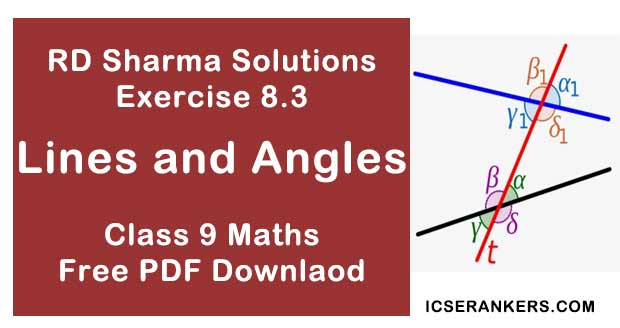Chapter Name RD Sharma Chapter 8 Lines and Angles Exercise 8.3 Book Name RD Sharma Mathematics for Class 10 Other Exercises Exercise 8.1Exercise 8.2Exercise 8.4 Related Study NCERT Solutions for Class 10 Maths

### Exercise 8.3 Solutions

1. In the below fig, lines l1 and l2 intersect at O, forming angles as shown in the figure. If x = 45, Find the values of x, y, z and u.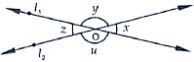Solution

Given that
x = 45° , y = ? , z = ?, u = ?
Vertically opposite sides are equal
∴ z = x = 45°
z and u angles are linear pair of angles
∴ z + u = 180°
z = 180° - 4
⇒ u = 180° - x
⇒ u = 180° - 45° [∵ x = 45°]
⇒ u = 135°
x and y angles are linear pair of angles
∴ x + y = 180°
y = 180° - x
⇒ y = 180° - 45°
⇒ y = 135°
∴ x = 45°, y = 135°, z = 135° and u = 45°

2. In the below fig, three coplanar lines intersect at a point O, forming angles as shown in the figure. Find the values of x, y, z and u.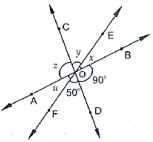Solution
Vertically opposite angles are equal
So ∠BOD = z = 90°
∠ DOF = y = 50°
Now, x + y + z = 180°  [Linear pair]
⇒ x + y + z = 180°
⇒ 90° + 50° + x = 180°
⇒ x = 180° - 140°
⇒ x = 40°

3. In the given fig, find the values of x, y and z.
From the given figure
∠y = 25° [∵ vertically opposite angles are equal]
Now
∠x + ∠y = 180° [Linear pair of angles are x and y]
⇒ ∠x = 180° - 25°
⇒ ∠x = 155°
Also
∠z = ∠x  155° [Vertically opposite angle]
∠y = 25°
∠x = ∠z = 155°

4. In the below fig, find the values of x.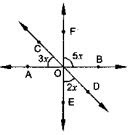Solution
Vertically opposite angles are equal
∠AOE = ∠BOF = 5x
Linear pair
∠COA + ∠AOE + ∠EOD = 180°
⇒ 3x + 5x + 2x = 180°
⇒ 10x = 180°
⇒ x = 18°

5. Prove that the bisectors of a pair of vertically opposite angles are in the same straight line.
Solution
Given,
Lines AOB and COD intersect at point O such that
∠AOC = ∠BOD
Also OE is the bisector ∠ADC and OF is the bisector ∠BOD
To prove : EOF is a straight line vertically opposite angles is equal
∠AOD = ∠BOC = 5x  ...(1)
Also ∠AOC + ∠BOD
⇒ 2∠AOE  = 2∠DOF ...(2)
Sum of the angles around a point is 360°
⇒ 2∠AOD + 2∠AOE + 2∠DOF = 360
⇒ ∠AOD + ∠AOF + ∠DOF = 180
From this we conclude that EOF is a straight line.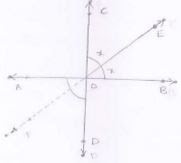Given that : - AB and CD intersect each other at O
OE bisects ∠COB
To prove: ∠AOF = ∠DOF
Proof: OE bisects ∠COB
∠COE = ∠EOB = x
Vertically opposite angles are equal
∠BOE = ∠AOF  = x  ...(1)
∠COE = ∠DOF = x  ...(2)
From (1) and (2)
∠AOF = ∠DOF = x

6. If one of the four angles formed by two intersecting lines is a right angle, then show that each of the four angles is  a right angle.
Solution
Given, AB and CD are two lines intersecting at O such that
∠ BOC = 90°
∠AOC = 90° , ∠AOD = 90° and ∠BOD = 90°
Proof:
Given that ∠BOC = 90°
Vertically opposite angles are equal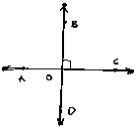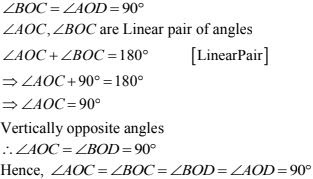7. In the below fig, rays AB and CD intersect at O.
(i) Determine y when x = 60°
(ii) Determine x when y = 40°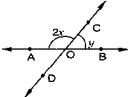Solution
(i) Given x = 60°
y = ?
∠AOC, ∠BOC are linear pair of angles
∠AOC + ∠BOC = 180°
⇒ 2x + y = 180°
⇒ 2×60° + y = 180°  [∵ x = 60°]
⇒ y = 180° - 120°
⇒ y = 60°
(ii) Given y = 40°, x = ?
∠AOC and ∠BOC are linear pair of angles
∠AOC + ∠BOC = 180°
2x + y = 180°
⇒ 2x + 40 = 180°
⇒ 2x = 140°
⇒ x = 140°/2
⇒ x = 70°

8.In the below fig, lines AB, CD and EF intersect at O. Find the measures of ∠AOC, ∠COF, ∠DOE and ∠BOF.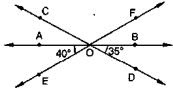Solution
∠AOE and ∠EOB are linear pair of angles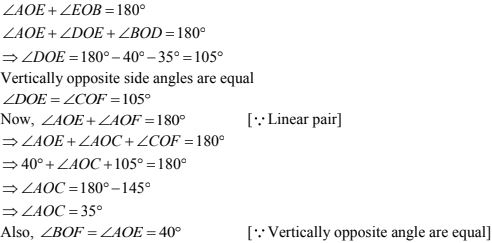9. AB, CD and EF are three concurrent lines passing through the point O such that OF bisects ∠BOD. If ∠BOF = 35 ,find ∠BOC and ∠AOD.
Solution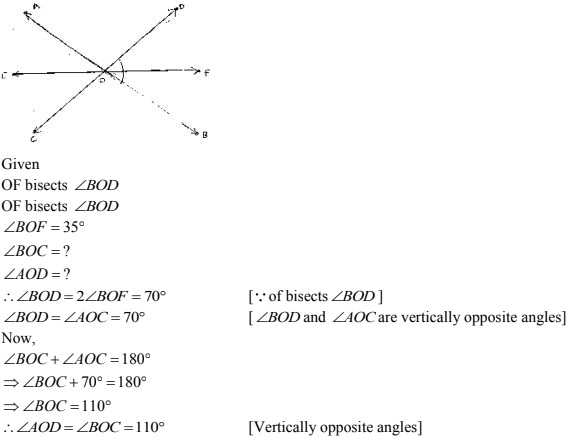10. In below figure, lines AB and CD intersect at O. If ∠AOC + ∠BOE = 70° and ∠BOD = 40°, find ∠BOE and reflex ∠COE.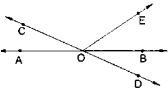Solution
Given that
∠AOC + ∠BOE = 70° and ∠BOD = 40°
∠BOE = ?
Here, ∠BOD and ∠AOC are vertically opposite angles
∠BOD = ∠AOC = 40°
Given ∠AOC + ∠BOE = 70°
40° + ∠BOF = 70°
∠BOF = 70°- 40°
∠BOE = 30°
∠AOC and ∠BOC are linear pair of angles
∠AOC + ∠COF + ∠BOE  = 180°
∠COE = 180° - 30° - 40°
⇒ ∠COE = 110°
∴ Reflex ∠COE = 360° - 110° = 250°.

11. Which of the following statements are true (T) and which are false (F) ?
(i) Angles forming a linear pair are supplementary.
(ii) If two adjacent angles are equal, and then each angle measures 90° .
(iii) Angles forming a linear pair can both the acute angles.
(iv) If angles forming a linear pair are equal, then each of these angles is of measure 90° .
Solution
(i) True
(ii) False
(iii) False
(iv)  True

12. Fill in the blanks so as to make the following statements true:
(i) If one angle of a linear pair is acute, then its other angle will be __________
(ii) A ray stands on a line, then the sum of the two adjacent angles so formed is ________.
(iii) If the sum of two adjacent angles is 180° , then the ________ arms of the two angles are opposite rays.
Solution
(i) Obtuse angle
(ii) 180°
(iii) uncommon